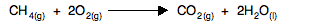Chemguide: Support for CIE A level Chemistry

```
```

Learning outcome 23: Chemical energetics

23.3: Entropy change, ΔS

```
```

Learning outcome 23.3.3

This statement is about calculating entropy changes for a reaction given values of standard entropies for everything taking part.

Before you go on, you should find and read the statement in your copy of the syllabus.

```
```

Standard entropies, S°

Entropy is given the symbol S, and standard entropy (measured at 298 K and a pressure of 1 bar) is given the symbol S°. You might find the pressure quoted as 1 atmosphere rather than 1 bar in less recent sources. Don't worry about it - they are nearly the same. 1 bar is 100 kPa; 1 atmosphere is 101.325 kPa. Use whatever units the examiners give you.

The units of entropy are J K-1mol-1. The thing you must be most careful about is the fact that entropy is measured in joules, not kilojoules, unlike most of the other energy terms you will have come across.

In an exam, you will be given values for all the standard entropies you need.

```
```

Entropy changes, ΔS°

Working out entropy changes for a reaction is very easy.

You add up the entropies for everything you end up with, and take away the entropies of everything you started with.

 Change in entropy = what you end up with - what you started with
```
```

Or if you like things mathematical:

ΔS° = ΣS°(products) - ΣS°(reactants)

Where Σ (sigma) simply means "the sum of".

```
```

In statement 23.3.2 we looked at the following reaction and worked out that there would be a decrease in entropy. Let's now do the calculation.CH4(g) O2(g) CO2(g) H2O(l) S° (J K-1mol-1) 186 205 214 69.9
```
```

You started with 1 mole of methane and 2 moles of oxygen.

Total starting entropy = 186 + 2(205) = 596 J K-1mol-1

You ended up with 1 mole of carbon dioxide and two moles of liquid water.

Total entropy at the end = 214 + 2(69.9) = 353.8 J K-1mol-1

Entropy change = what you end up with - what you started with.

Entropy change = 353.8 - 596 = -242.2 J K-1mol-1

Notice that it is a negative value. The entropy has decreased - as we predicted it would in the earlier statement. That's because there is a decrease in the total number of gas molecules present.

```
```

And that is all there is to it! You will, of course, need to practise doing this until you are completely confident, but you will need to find your own examples. There are lots in my calculations book if you have a copy. If you look at those, you can ignore any reference to the word "system" - CIE doesn't need this.

```
```

Go to the Section 23 Menu . . .